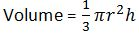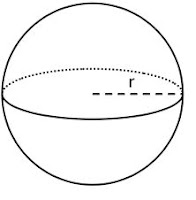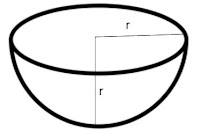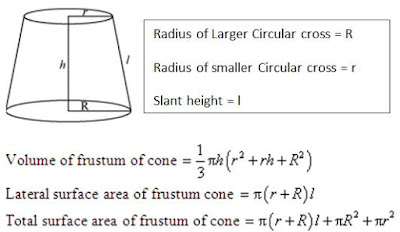## Revision Notes for Ch 13 Surface Areas and Volumes Class 10th Mathematics

Formulae for Curved Surface Area, Surface Area and Volume of Cuboid• Curved/lateral Surface Area = 2h(l +b)

• Surface Area = (lb+bh+lh)

• Volume = lbh

Formulae for Curved Surface Area, Surface Area and Volume of CubeSide of cube = a

• Curved/lateral surface area = 4a2

• Total Surface Area = 6a2

• Volume = a3

Formulae for Curved Surface Area, Surface Area and Volume of Cylinderh = Height of Cylinder

• Curved Surface Area = 2πrh

• Total Surface Area = 2πrh +2 πr2

• Volume = πr2h

Formulae for Curved Surface Area, Surface Area and Volume of Cylinderh= height of cone

l = slant height of cone =(h2+r2)

• Curved Surface Area = πrl

• Total Surface Area = πrl + πr2h

•Formulae for Surface Area and Volume of Sphere• Surface Area = 4πr2

•Formulae for Curved Surface Area, Total Surface Area and Volume of Hemi-Sphere